### Answers − Straight Line Graphs

#### 1.   Plot the graph y = 5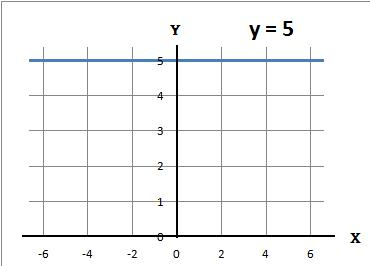#### 2.   Plot the graph y = x − 3

(a) Using a table work out the values of y

 x −1 0 1 y = x − 3 −4 −3 −2

(b) The coordinates of the above are (−1, −4) (0, −3) and (1, −2)

(c) Plot the above coordinates and draw a line through these points#### 3.   Plot the graph y = −x

(a) Using a table work out the values of y

 x −1 0 1 y = −x 1 0 −1

(b) The coordinates of the above are (−1, 1) (0, 0) and (1, −1)

(c) Plot the above coordinates and draw a line through these points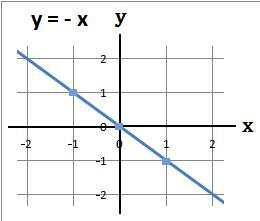#### 4.   Plot the graph y = −2 − x

(a) Using a table work out the values of y

 x −1 0 1 y = −2 − x −1 −2 −3

(b) The coordinates of the above are (−1, −1) (0, −2) and (1, −3)

(c) Plot the above coordinates and draw a line through these points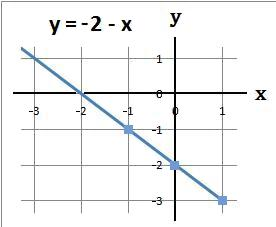#### 5.   Plot the graph y = 3x + 4

(a) Using a table work out the values of y

 x −1 0 1 3x −3 0 3 +4 +4 +4 +4 y = 3x + 4 1 4 7

(b) The coordinates of the above are (−1, 1) (0, 4) and (1, 7)

(c) Plot the above coordinates and draw a line through these points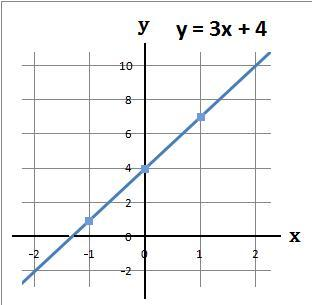#### 6.   Plot the graph y = 3 − 2x

(a) Using a table work out the values of y

 x −1 0 1 3 3 3 3 −2x 2 0 −2 y = 3 − 2x 5 3 1

(b) The coordinates of the above are (−1, 5) (0, 3) and (1, 1)

(c) Plot the above coordinates and draw a line through these points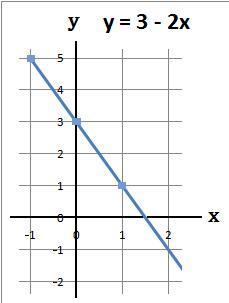#### 7.   Plot the graph 2y − x = 5

(a) Make y the subject

 2y − x = + 5 + x = + x note (1)
 2y = x + 5 ÷ 2 = ÷ 2 note (2)
 y = (x + 5)/2

Note: (1) add x to both sides
Note: (2) divide both sides by 2

(b) Using a table work out the values of y

 x −1 0 1 x + 5 4 5 6 ÷2 ÷2 ÷2 ÷2 y = (x + 5)/2 2 2.5 3

(c) The coordinates of the above are (−1, 2) (0, 2.5) and (1, 3)

(d) Plot the above coordinates and draw a line through these points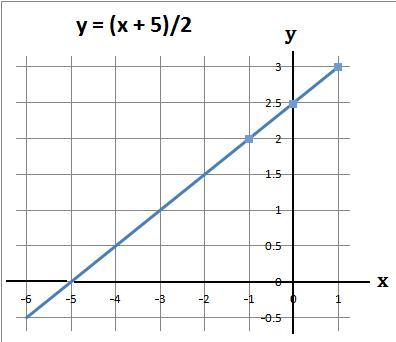back to: